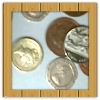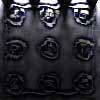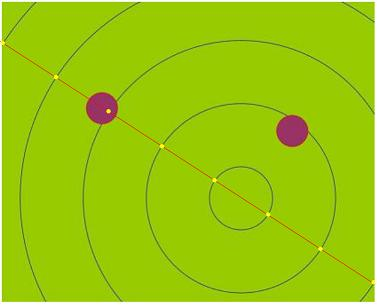#### You may also like### Win or Lose?

A gambler bets half the money in his pocket on the toss of a coin, winning an equal amount for a head and losing his money if the result is a tail. After 2n plays he has won exactly n times. Has he more money than he started with?### Fixing the Odds

You have two bags, four red balls and four white balls. You must put all the balls in the bags although you are allowed to have one bag empty. How should you distribute the balls between the two bags so as to make the probability of choosing a red ball as small as possible and what will the probability be in that case?### Scratch Cards

To win on a scratch card you have to uncover three numbers that add up to more than fifteen. What is the probability of winning a prize?

# Coin Lines

##### Age 14 to 16Challenge Level

The person who sent in this solution didn't add their name, but it's a nice visualisation.

To start off, consider the situation where the distance between the two lines is double the diameter of the coin. Call the diameter of the coin d - so we can say the centre of the coin is 0.5d from the edge of the coin. The distance between the lines is 2d. For the coin not to cross either line, the centre of the circle must be a perpendicular distance of between 0.5d and 1.5d (inclusive) away from a line. The area between the two lines then "allowed" for the centre of the coin to land in without touching a line is therefore half of the total area between the two lines - so assuming the centre of the coin is equally likely to land in all areas, the coin will touch a line half the time.

Now a good way to think about the concentric circles is to imagine the coin somewhere and focus on a line through the centre of the coin and the centre of the concentric circles.The situation is the same as the one with straight lines I have considered already. So the answer is 0.5 or d, if the gap is 1 and the coin diameter is d.